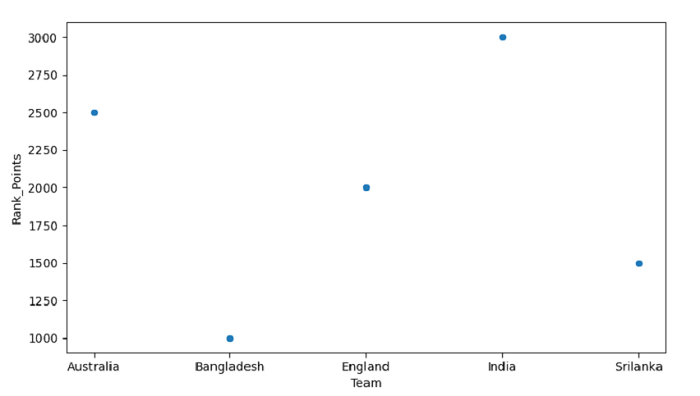# Python - Draw a Scatter Plot for a Pandas DataFrame

Scatter Plot is a data visualization technique. Use the plot.scatter() to plot the Scatter Plot. At first, Let us import the required libraries −

We have our data with Team Records. Set it in the Pandas DataFrame −

data = [["Australia", 2500],["Bangladesh", 1000],["England", 2000],["India", 3000],["Srilanka", 1500]]

dataFrame = pd.DataFrame(data, columns=["Team","Rank_Points"])



Let us plot now with the columns −

dataFrame.plot.scatter(x="Team", y="Rank_Points")

## Example

Following is the code −

import pandas as pd
import matplotlib.pyplot as mp

# our data
data = [["Australia", 2500],["Bangladesh", 1000],["England", 2000],["India", 3000],["Srilanka", 1500]]

# dataframe
dataFrame = pd.DataFrame(data, columns=["Team","Rank_Points"])

# scatter plot the dataframe
dataFrame.plot.scatter(x="Team", y="Rank_Points")

# displaying scatter plot
mp.show()

## Output

This will produce the following output